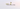# How to Convert Array to a String in JavaScriptIn this tutorial, we are going to learn about converting an array to a string in JavaScript.

## First way: Using join() method

The `join()` method converts the array of elements into a string separated by the commas.

Example:

``````const arr = [1,'a','b','c'];

console.log(arr.join());

// output --> "1,a,b,c"``````

We can also pass our own `separator` as an argument to the `join()` method.

In this below example, we passed `-` as a separator argument so that string is separated by a minus operator `-`.

``````const arr = [1,'a','b','c'];

console.log(arr.join('-'));

// output --> "1-a-b-c"``````

## Second Way: Using `toString()` method

The `toString()` method also joins the array and returns the string where the array of elements is separated by the commas.

Example:

``````const arr = [1,'a','b','c'];

console.log(arr.toString());

// output --> "1,a,b,c"``````

## Third way: Using an empty string

If we add an array with an empty string `''` it converts the array to a string.

Example:

``````const arr = [1,'a','b','c'];

console.log(arr + '');

// output --> "1,a,b,c"``````

## Top Udemy Courses##### JavaScript - The Complete Guide 2020 (Beginner + Advanced)
45,614 students enrolled
52 hours of video content
View Course##### React - The Complete Guide (incl Hooks, React Router, Redux)
284,472 students enrolled
40 hours of video content
View Course##### Vue - The Complete Guide (w/ Router, Vuex, Composition API)
130,921 students enrolled
48.5 hours of video content
View Course